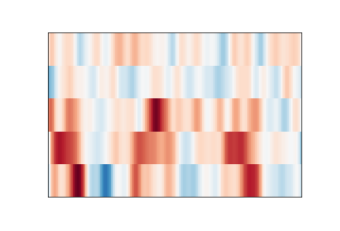# Get rid of external white spaces from matplotlib plot

I want to get rid of white spaces from this plot. The plot is from `mne` library example here. I only want to data in the box and nothing else. I converted the plot to image like this:

``````def get_img_from_fig(fig, dpi=180):
buf = io.BytesIO()
fig.savefig(buf, format="png", dpi=fig.dpi)
buf.seek(0)
img_arr = np.frombuffer(buf.getvalue(), dtype=np.uint8)
buf.close()
img = cv2.imdecode(img_arr, 1)
img = cv2.cvtColor(img, cv2.COLOR_BGR2RGB)
return img
``````I tried a lot of things including this:

``````plt.axis('off')
plt.imshow(img_orig_2)
plt.savefig('test.png', bbox_inches='tight',pad_inches = 0, dpi = 180)
plt.show()
``````

but it won’t get rid of the white space. What should I do besides manually cropping or setting the aspect ratio?

My current recipe is this `remove_decorations` function:

I have no idea if it is sufficiently complete. You have to do this
after all the plotting but before you show or save the plot.

Cheers,
Cameron Simpson cs@cskk.id.au

···

On 18Sep2022 05:29, Flock-Anizak via Matplotlib nobody@discourse.matplotlib.org wrote:

I want to get rid of white spaces from this plot. The plot is from
`mne` library example
here.
I only want to data in the box and nothing else. […]

1 Like

Is there a way I can do this in python? How do I use this when I’m training a machine learning model?

That function is is Python. Here’s an example use of it:

Ignoring the larger programme, the line:

`````` figure = spd.plot(.......)
``````

returns a matplotlib `Figure` containing a plot of my solar inverter
data. The next statement:

`````` if bare:
remove_decorations(figure)
``````

removes the decoarations from the figure if that mode has been selected.
And the lines below that save or display the figure.

`````` def get_img_from_fig(fig, dpi=180):
buf = io.BytesIO()
fig.savefig(buf, format="png", dpi=fig.dpi)
.....
``````

appears to my eye to save a `Figure` to a `BytesIO` instance. You’d call
`remove_decorations(fig)` just before `fig.savefigure(...)`.

As I understand matplotlib’s model, a `Figure` is a representation of
all the plots you’ve issued, but that representation has not been
“drawn” and is not drawns until you show or save the `Figure`. That
means you can manipulate the `Figure` to remove decoations like legends
of axis markings. Because legends and axis markers are created by
automatically during various plotting operations, you need to do this
after the plotting but before the show or save.

Cheers,
Cameron Simpson cs@cskk.id.au

···

On 18Sep2022 17:50, Flock-Anizak via Matplotlib nobody@discourse.matplotlib.org wrote:

Is there a way I can do this in python? How do I use this when I’m
training a machine learning model?